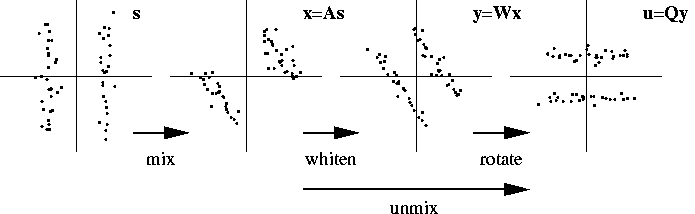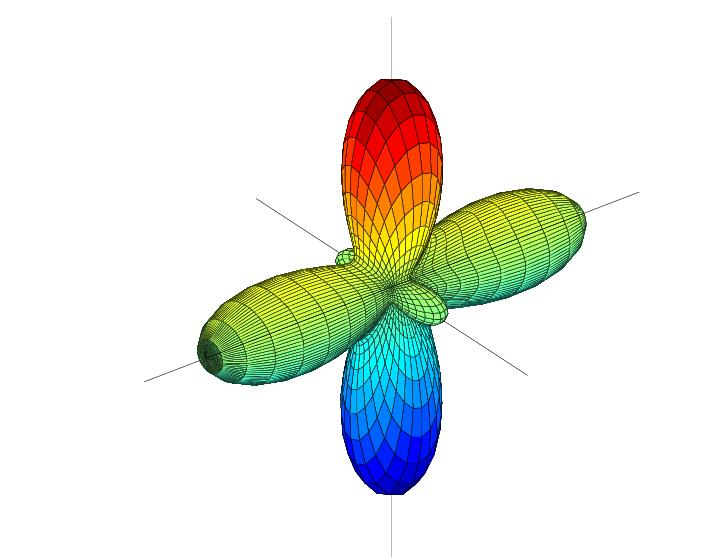Research Project (2001, 2002)

# Improved cumulant based method for independent component analysis

Tobias Blaschke and Laurenz Wiskott

Independent component analysis (ICA) is a linear technique for performing blind source separation, i.e. for unmixing a linear mixture of statistically independent sources; see Figure 1. One popular class of ICA-algorithms is based on cumulants, which are descriptors of statistical moments of data distributions. If several signals are statistically independent, then all mixed cumulants, i.e. those involving two or more signals, vanish. Cumulant-based ICA-Algorithms now work the other way around. They try to find a linear unmixing that minimizes the absolute values of all mixed cumulants, in other words the cumulant-tensors are diagonalized.Figure 1: Linear mixing and unmixing of two statistically independent sources. The unmixing is done in two steps. Whitening diagonalizes the second-order cumulant-tensor. Then actual ICA is applied and performs a rotation that diagonalizes also higher-order cumulant-tensors. Unmixing is determined only up to a scaling and permutation of the sources.

There are no mixed first-order cumulants and second-order cumulant-tensors can always be diagonalized exactly by whitening without actually separating the sources. Thus it is the higher-order cumulants that matter. Typically only fourth-order cumulants are taken into account, which means that the algorithms are blind to other than fourth-order statistics. In this project we have developed an algorithm for performing Cumulant Based ICA (= CuBICA) that combines third- and fourth-order cumulants. It is therefore able to separate symmetric as well as skew-symmetric sources simultaneously without any parameter adjustments. The objective function is also mathematically simpler than those of earlier algorithms. The table shows a performance comparison between different algorithms on different data sets. CuBICA performs well on all data sets and requires no parameter tuning at all.

 unmixing error CPU time on data set (.) with N components on data set (.) with N components (i) (ii) (iii) (iv) (v) (i) (ii) (iii) (iv) (v) N=6 N=6 N=7 N=12 N=40 N=6 N=6 N=7 N=12 N=40 CuBICA 0.017 0.039 0.041 0.038 0.039 1.5 1.4 2.8 10.1 230.3 Comon 0.017 0.25 0.14 0.049 0.061 2.4 2.3 4.3 14.1 300.2 JADE 0.016 0.30 0.11 0.035 0.10 0.7 0.7 1.1 5.4 404.6 Infomax 0.018 0.47 0.17 0.043 0.035 48.1 49.3 57.8 112.1 512.3 FastICA 0.016 0.040 0.11 0.042 0.037 1.7 0.5 0.5 6.2 16.8

Table: Performance comparison between different ICA-algorithms on different data sets. On the left is shown the unmixing error (see the publications below for a definition) and on the right the CPU times [sec] used by the algorithms. Good performances are shown in bold face. CuBICA, Comon's algorithm, and JADE required no parameter tuning. For FastICA different non-linearities had to be chosen for different data sets to get the results shown in the table. Infomax required a lot of parameter tuning to make it converge properly. the CPU-time of Infomax only refers to the final run and does not include the time used for parameter tuning. Data set (ii) contained five skew-normally distributed sources; data set (iii) was a mixture of three music sources and three skew-normally distributed sources plus one Gaussian. The presence of skew-normally distributed sources is the reason why CuBICA performs so well compared to the other algorithms. Only FastICA was able to also unmix data set (ii) because an appropriate non-linearity could be chosen manually. The number of data points for all data sets was T=44218.

The Matlab source code of CuBICA can be downloaded from the web pages of Tobias Blaschke.

As a side issue we have also thought about how to visualize the objective function and Figure 2 shows what we came up with.Figure 2: Visualization of a contrast function in three dimensions. The surface shows the value of the kurtosis squared in all different directions of the 3-dimensional space. Since minimizing the mixed fourth-order cumulants is equivalent to maximizing the squared kurtosis of the output signal components, cumulant based ICA tries to find three orthogonal axes (indicated by thin lines) along which the displayed surface has maximal total distance from the origin.

See also the slides of the talk I gave at the European Summer School on ICA - from Theory to Applications.

### Relevant Publications:

Black colored reference are the principal ones. Gray colored references are listed for the sake of completeness only. They contain little additional information. .ps-files are optimized for printing; .pdf-files are optimized for viewing at the computer.

1. Blaschke, T. (25. May 2005).
Independent component analysis and slow feature analysis.
Doktorarbeit, Institut für Physik, Humboldt-Universität zu Berlin, Germany.
(bibtex, abstract.html, paper)

2. Blaschke, T. and Wiskott, L. (May 2004).
CuBICA: Independent component analysis by simultaneous third- and fourth-order cumulant diagonalization.
IEEE Transactions on Signal Processing, 52(5):1250-1256.
(bibtex, abstract.html, paper.pdf, paper.ps.gz)

3. Blaschke, T. and Wiskott, L. (9. April 2003).
CuBICA: Independent component analysis by simultaneous third- and fourth-order cumulant diagonalization.
Computer Science Preprint Server (CSPS) http://www.sciencedirect.com/preprintarchive: Computational Intelligence/0304002.
(bibtex, abstract.html)

4. Blaschke, T. and Wiskott, L. (27. August 2002).
An improved cumulant based method for independent component analysis.
Proc. Int'l Conf. on Artificial Neural Networks, ICANN'02, Madrid, August 27-30, ed. José R. Dorronsoro, in series Lecture Notes in Computer Science, publ. Springer-Verlag, pp. 1087-1093.
(bibtex, abstract.html)

setup April 24, 2002; updated August 26, 2005
Laurenz Wiskott, http://www.neuroinformatik.ruhr-uni-bochum.de/PEOPLE/wiskott/